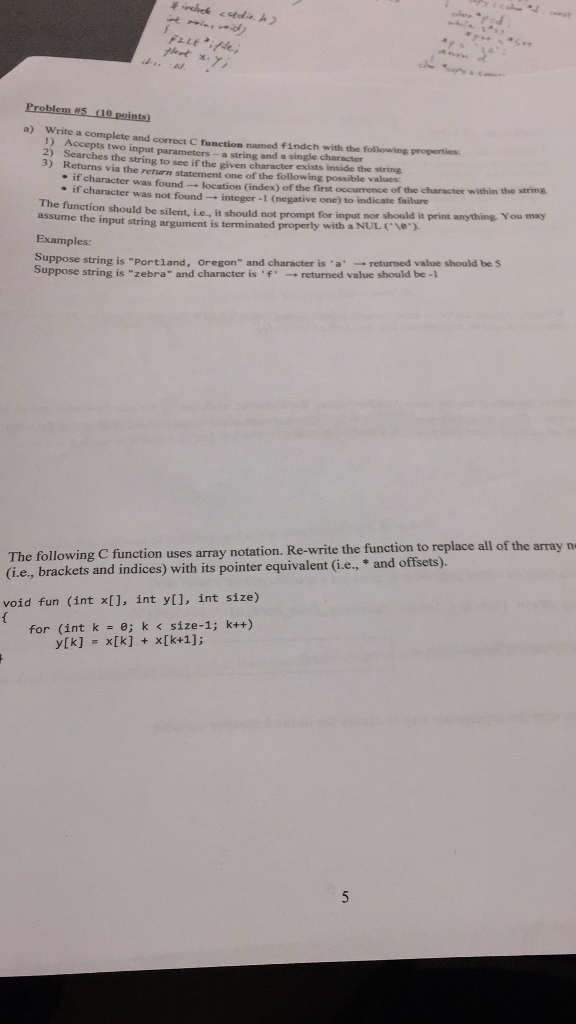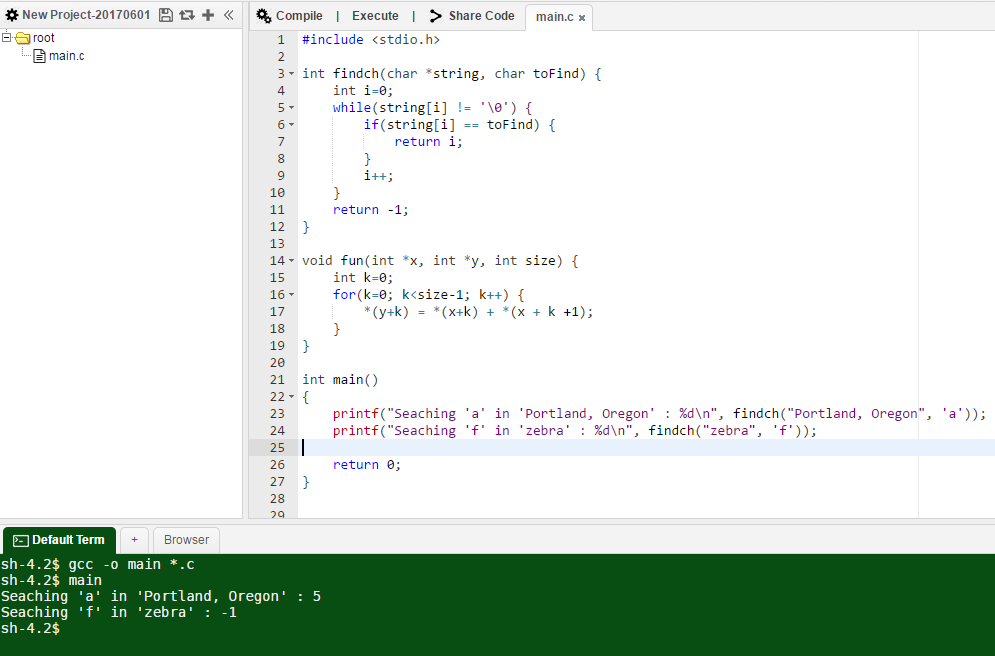# Answered! a) Write a complete and correct C function named findch with the following properties. 1) Accepts two parameters-a…Write a complete and correct C function named findch with the following properties:…

Don't use plagiarized sources. Get Your Custom Essay on
Answered! a) Write a complete and correct C function named findch with the following properties. 1) Accepts two parameters-a…
GET AN ESSAY WRITTEN FOR YOU FROM AS LOW AS \$13/PAGE

a) Write a complete and correct C function named findch with the following properties. 1) Accepts two parameters-a string and a single character Searches the string to see if the given character exists inside the string Returns via the return statement one of the following possible values: if character was found rightarrow location (index) of the first occurrence of the character within the string if character was not found rightarrow integer -1 (negative one) to indicate failure The function should be silent, i.e., it should not prompt for input nor should in print anything. You may assume the input string argument is terminated properly with a NUL ( ) Suppose string is “Portland, Oregon” and character is a rightarrow returned value should be 5 Suppose string is “zebra” and character is ‘f’ rightarrow returned value should be -1 The following C function uses array notation. Re-write the function to replace all of the array (i.e., brackets and indices) with its pointer equivalent (i.e., *and offsets). void fun (int x[], int y[], int size) { for (int k = 0:k size-1 k++) y[k] = x[k] + x[k+1];

#include <stdio.h>

int findch(char *string, char toFind) {
int i=0;
while(string[i] != ‘’) {
if(string[i] == toFind) {
return i;
}
i++;
}
return -1;
}

void fun(int *x, int *y, int size) {
int k=0;
for(k=0; k<size-1; k++) {
*(y+k) = *(x+k) + *(x + k +1);
}
}

int main()
{
printf(“Seaching ‘a’ in ‘Portland, Oregon’ : %dn”, findch(“Portland, Oregon”, ‘a’));
printf(“Seaching ‘f’ in ‘zebra’ : %dn”, findch(“zebra”, ‘f’));

return 0;
}